﻿ Harmonic Aggregation Techniques: Methods to Compensate for Interaction Effects

Harmonic Aggregation Techniques: Methods to Compensate for Interaction Effects

Mohammad Mahdi Share PasandDepartment of Electrical and Electronics Engineering, Standard Research Institute- SRI, Alborz, Iran

Abstract

The interaction effect in aggregation of harmonic currents is investigated. Several approaches including IEC 61000-3-6 recommended method are utilized and compared. A simple practical method is proposed to account for/compensate interaction effect. The proposed technique is computationally simple and does not require the knowledge of current harmonics in each branch. A rectifier is considered and simulated as an example to show the interaction effect and performance of the proposed method.

• Mohammad Mahdi Share Pasand. Harmonic Aggregation Techniques: Methods to Compensate for Interaction Effects. American Journal of Electrical and Electronic Engineering. Vol. 3, No. 3, 2015, pp 83-87. http://pubs.sciepub.com/ajeee/3/3/4
• Pasand, Mohammad Mahdi Share. "Harmonic Aggregation Techniques: Methods to Compensate for Interaction Effects." American Journal of Electrical and Electronic Engineering 3.3 (2015): 83-87.
• Pasand, M. M. S. (2015). Harmonic Aggregation Techniques: Methods to Compensate for Interaction Effects. American Journal of Electrical and Electronic Engineering, 3(3), 83-87.
• Pasand, Mohammad Mahdi Share. "Harmonic Aggregation Techniques: Methods to Compensate for Interaction Effects." American Journal of Electrical and Electronic Engineering 3, no. 3 (2015): 83-87.

 Import into BibTeX Import into EndNote Import into RefMan Import into RefWorks

1
Prev Next

1. Introduction

Power quality has been attracting increasing attention during recent years. This is mostly due to increasing use of nonlinear power electronic/ switching devices. On the other hand, much more number of sensitive electronic components such as computers is being used each day. This results in a growing importance of power quality issues. Harmonic pollution (i.e. levels of high order harmonics in the current/voltage waveform with a magnitude which causes unacceptable performance degradation) may cause compatibility problems between different components connected to a shared bus, distribution losses, damage to several components such as transformers, power switches and electric motors, accidental operation of remotely controlled switches and breakers(false tripping), equipment malfunction due to excess voltage, metering errors in power distribution and distributed measurement and control systems, fires in wiring, penalties on monthly bill units, generator failures etc. Permissible levels of harmonic pollution in IEC and IEEE international standards are provided as static indices measured at PCCs {1}[3, 4]. However, since it is not always practical to measure power quality indices in PCCs, especially when the network behavior is time varying (e.g. inserting new loads, time varying components, etc.), some approximate methods are recommended in IEC 61000 series for evaluating the total harmonic current based on single component behavior .

To have a proper estimation of harmonic current based on individual harmonic currents is that one can determine whether it is possible to add new components without violating the THD requirements or not. If the new component violates the THD level requirements, it has to be integrated with a harmonic filter. Also in the situation when one or a few loads are to be provided with a harmonic filtering means to reduce THD, it is desired to be able to determine the harmonic level after adding the filter at the design stage. Also appropriate aggregation formulae will be useful for optimal placement of harmonic filters in a network. (i.e. whether to provide each load with its harmonic filter or to design a single filter for a group of loads consisting n loads, and to determine n to yield best results).

Two major phenomena may impact aggregation of harmonic currents. First is the magnitude aggregation error which is caused by the phase differences between several harmonic components. This issue has been addressed in [1, 2, 3, 4] and references therein. Another complication which is to be addressed in this paper is the interaction effect. Interaction is the effect of adding new loads on the harmonic components of the previously installed loads. This phenomenon is caused because of line impedance which results in harmonic voltages due to harmonic currents. As a result, a nonlinear load could pollute the distribution network via line impedances carrying harmonic currents. Interaction effect has not been issued in previous works, since the common de-facto method for harmonic aggregation studies is to model harmonic loads as harmonic current sources. [8, 9, 10, 13, 14] However in real world, harmonic loads may exhibit different harmonic currents depending on applied voltages. As a result, a load drawing harmonic current may cause another load to draw more harmonic current through harmonic voltage drop in the line.

2. Approximation of Harmonic Current

The most conservative method to sum up individual harmonics is to add the harmonic currents magnitudes (peak or RMS) to estimate the aggregated harmonic current. This method, namely linear summation, presumes that alls are in phase as stated in equation (1). If the components (usually load currents) are in phase, a scalar summation would be accurate; however this will lead to a conservative yet unrealistic approximation of THD . It has been represented that in many cases, arithmetic summation is too pessimistic and actual harmonics cancels each other due to phase differences, resulting in a very smaller aggregated harmonic level. (See  and references therein.)

Another method is to vector-sum the harmonic currents which lead to an exact value for the aggregated harmonic current. This method is accurate, however the actual phases are not constant  and the so called vector sum may result inaccurate estimation if the phase angles are not measured for a long enough period. An approximate method is to use statistical methods as  which require a probability density function for harmonic phases or sums. The probability that a phase equals a specific value depends on switching time which is human dependant as well as internal circuitry of the device.

The third method is to establish formulae based on empirical data. IEC 61000-3-6 recommends to add up harmonics based on Table 2 and equation 2, which is arithmetic summation for harmonic orders below 5 and a root of sums for higher orders (2).(1)

In which the following notation is used: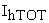: Aggregated harmonic current (order h) for all components: Harmonic current (order h) for jth component

IEC 61000 recommended formulation is:(2)

In which:

Table 1. IEC 61000-3-6 method for harmonic aggregationDownload asVeiw tableTables index

Using root sum of squares –RSS– is also possible, assuming 90 degrees phase differences. Other aggregation techniques include summation of vector components presuming random phase differences driven by probability densities of different types. These methods require experimental data to estimate phase angle difference PDF, from the experimental histograms. Statistical approaches are more logical to be applied especially when an appropriate density function is available. Actual PDFs can be modeled only through complicated analytical functions.  In addition, because of fixed limits recommended for THD levels [4, 9], it is more practical to derive conservative deterministic models based on statistical analysis. One approach is to define a percentile in the statistical model, not to be violated by the system. For instance  sets 5% limit for the THD (i.e. The THD level may not exceed 95% of the recommended limit) which is recommended by IEC as well .

The recommended methods –namely harmonic aggregation techniques or methods of harmonic summation are not accurate due to the fact that harmonic components have different- usually time variant - phases. A statistical analysis of harmonic phases is performed in , in which probability density functions are estimated on the basis of simplifying assumptions. The PDF of harmonic phases is considered to be uniform in [6, 7], which are too optimistic. In fact, the statistical analysis of harmonics is valid only if experimental data for individual appliance harmonics is used to model phase behaviors. In this study, experimental data are drawn to model the phase behavior, examine the current aggregation method, evaluate the statistical assumptions of  and to derive statistical indices for THD levels which is optimistically considered to be in steady state in standards IEEE 519, IEC 61000 (CIGRE is one exception  which admits that permissible harmonics could be time varying).

3. Interaction Effects on Aggregated and Individual Harmonic Currents

Definition: Interaction drift is defined as follows:In which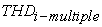represents the total harmonic distortion of current drawn by a load paralleled with a number of identical loads and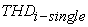represents the total harmonic distortion of current drawn by a single load connected directly to the voltage source.

Interaction drift is caused because of the voltage drop between parallel loads due to resistance / impedance existing in wirings. It may also model the change in harmonic distortion due to current reduction in each load being paralleled with other loads.Download asVeiw figureFigures index
Figure 1. Harmonic spectrum of a rectifier

Consider N identical loads with similar phase behaviors. A proper example of this situation is a bank of paralleled voltage controlled switching devices like a bank of rectifiers. In this situation, phase of harmonic current does not affect the aggregated current and therefore the interaction effect could be analyzed independently.

Figure 1 depicts harmonic components of a rectifier.

A line impedance of 0.1Ω is considered to model line impedance and its effect on individual harmonic levels.

Table 2. THD levels for different number of loadsDownload asVeiw tableTables index

As shown by Table 2, increasing the number of identical loads, does not severely affect the THD level. This is due to the fact that harmonic currents of this type of load have almost equal phases in all times. The following figure depicts phase behavior of half wave rectifiers.Download asVeiw figureFigures index
Figure 2. Phase behavior of 5th harmonic current in a rectifier

Unlike THD level, current harmonics are affected when additional loads are inserted into the bus. The flow of harmonic currents through line impedance induces harmonic voltage drops and causes harmonic pollution to distribute through the network. This along with the first harmonic voltage drop causes a load to draw different current compared to the situation in which a single load is directly connected to the bus. The following table depicts the difference cause by interaction effect. Interaction effect is of great importance especially when power electrical loads are located far from each other and line impedances are significant.

Table 3. Current Harmonics of order 3, for different number of loadsDownload asVeiw tableTables index

It is not practical to measure harmonics of all branches, therefore it is desired to estimate total harmonic current based on harmonic current of a single load and phase behavior of loads. Practical approximation (left column of Table 3 and Table 4) is based on the measured current harmonic for a single load which could be approximated from THD level or as a worst case scenario, by the fact that a single device complies with an emission EMC standard as in .

In Table 4, two approximate values are included due to the difference between approximations given in Table 1 for harmonic orders below and above 5.

- Harmonic currents of diode rectifiers are mostly in phase and summation method is the most appropriate estimation. This is due to the fact that change in forward voltages of diodes does not impact the phase of harmonic currents. (Phase difference is about 0.001rad per 1volt forward voltage difference).

- In presence of line resistance, harmonic currents of loads are generally decreased. Therefore, estimation of total current harmonic based on harmonic current of a single load is not accurate. (Compare 3rd and 7th columns of Table 3 and Table 4)

Assuming identical loads with slight differences in phase and magnitude of harmonics, one may obtain the following approximate relations:

- As line impedance affects harmonic currents, jth harmonic current of the kth branch could be approximated as: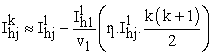In whichand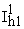represent the jth and 1st harmonic currents of a single load.

Table 4. Current Harmonics of order 5, for different number of loadsDownload asVeiw tableTables index

- The aggregated harmonic current for N branches, could be therefore computed as:Where loads do not exhibit similar phase behavior, the aforementioned approximation will be too conservative and the first component of approximation could be replaced considering appropriate approximation methods like the one given in .

The advantage of this proposed method is that it does not require any measurement in individual branches. The only measurements needed are the first and jth harmonic current components, number of similar branches (loads or PCCs) and bus voltage.

The following tables compare practical approximations for aggregated current harmonic in 3rd and 5th harmonic orders.

Table 5. Comparison of practical approximation methodsDownload asVeiw tableTables index

Table 6. Comparison of practical approximation methodsDownload asVeiw tableTables index

The following figure shows the approximations and actual values of aggregated harmonic current. The proposed method is situated above the actual value when the number of loads increases. Note that using approximate values is inevitable in most applications because of practical limitations in measuring all harmonic values.Download asVeiw figureFigures index
Figure 3. Practical approximations for aggregated 5th harmonic current

Figure 3 shows that the proposed method results in acceptable aggregated value which is below the actual value for small number of loads. Because loads are similar, the best approximation for the aggregated harmonic is linear summation; however, linear summation requires the value of harmonic currents in all branches which is not practical. Other methods including RSS and alpha approximation are less accurate as there is no cancellation due to phase difference in harmonic currents of this example.

4. Conclusion

The interaction effect which may adversely impact the accuracy of harmonic aggregation computations is studied. It is shown that existing approximations are not accurate or require extensive measurement data to calculate the aggregated harmonic. A formulation is proposed for a set of similar nonlinear loads to calculate the aggregated harmonic accurately and without need to measure harmonic currents at each branch. Examples are investigated with in-phase harmonic loads using different methods including IEC 61000-3-6 aggregation formulae.

Notes

1. Point of Common Coupling

2. Current Harmonic

3. Based on IEC 61000-3-6 given in Table 1

4. Based on IEC 61000-3-6 for h<5

5. Based on IEC 61000-3-6 for h>5

References

  Allen, George W, “Design of Power-Line Monitoring Equipment”, IEEE Transactions on Power Apparatus and Systems, Vol.PAS-90, Issue: 6, Nov. 1971.In article View Article  S Chattopadhyay, M Mitra, S Sengupta , “Electric power quality”, Springer, 2011.In article View Article  G.D.Castro, A., M. Bollen and A. Moreno-Monuz, “Street Lamps Aggregation Analysis Through IEC 61000-3-6 Approach”, International Conference on Electricity Distribution, Stockholm, June 2013.In article View Article  Pasand, Mohammad Mahdy Share, and Zahra Rahmatian. “Harmonic aggregation techniques for power quality assessment a standard framework.” International Journal of Engineering & Technology 3.3 (2014): 365-371.In article  Pasand, MM Share. “Harmonic Aggregation Techniques for Power Quality Assesment A review of different methods.” International Journal of Engineering Science and Technology 6.7 (2014): 423.In article  IEEE std. 519, “Recommended Practices and Requirements for Harmonic Control in Electrical Power Systems”, 1992.In article  IEC 61000, “Electromagnetic compatibility (EMC) – Part 3-6: Limits – Assessment of emission limits for the connection of distorting installations to MV, HV and EHV power systems”, Basic EMC publication, ed.2, 2008. (IEC/TR 61000-3-6).In article  Y. Baghzouz, et.al., “Time-Varying Harmonics: Part II—Harmonic Summation and Propagation” , IEEE Transactions On Power Systems, Vol. 17, No. 1, 2002.In article View Article  Y. Baghzouz, Task Force on Probabilistic Aspects of Harmonics: “Time-varying harmonics: Part I—Characterizing measured data,” IEEE Trans. Power Delivery, vol. 13, pp. 938-944, 1998.In article View Article  A. Cavallini, R. Langella, et.al., “Gaussian modeling of harmonic vectors in power systems,” in IEEE 8th Int. Conf. Harmonics and Quality of Power, Athens, Greece, Oct. 14–16, 1998, pp. 1010-1017.In article View Article  IEC 61000‐3‐2: 2009, Electromagnetic Compatibility (EMC) - Part 3-2: Limits-Limits for harmonic current emissions (Equipment input current ≤ 16A per phase) (IEC 61000-3-2).In article  CIGRE WG 36-05, “Assessing voltage quality with relation to harmonics, flicker and unbalance,”, France, CIGRE Rep. 36-203, 34th Session, 1992.In article  Medeiros, F. ; Brasil, D.C. ; Ribeiro, P.F. ; Marques, C.A.G. ; Duque, C.A. “A new approach for harmonic summation using the methodology of IEC 61400-21”, 14th International Conference on Harmonics and Quality of Power (ICHQP), 2010.In article View Article  Hsiao, Y. “Design of Filters for Reducing Harmonic Distortion and Correcting Power Factor in Industrial Distribution Systems.” Tamkang Journal of Science and Engineering, 4(3), 193-199, 2001.In article  Wakileh, G. J. “Power systems harmonics: fundamentals, analysis, and filter design”, Berlin: Springer, 2001.In article View Article  Persson, Jonas, “Comparing Harmonics Mitigation Techniques.” (2014).In article  Buccella, Concettina, et al. “Harmonic mitigation technique for multilevel inverters in power systems.” Power Electronics, Electrical Drives, Automation and Motion (SPEEDAM), 2014 International Symposium on. IEEE, 2014.In article View Article  Aye, Thet Mon, and Soewin Naing. “Analysis of Harmonic Reduction by Using Passive Harmonic Filters.” (2014).In article  Sharma, Vandana, and Anurag Singh Tomer. “Comparative Analysis on Control Methods of Shunt Active Power Filter for Harmonics Mitigation.” International Journal of Science and Research 3 (2014).In article  Yang, Kai, Math HJ Bollen, and E. O. Anders Larsson. “Aggregation and amplification of wind-turbine harmonic emission in a wind park.” Power Delivery, IEEE Transactions on 30.2 (2015): 791-799.In article  Yang, Kai, Math HJ Bollen, and E. O. Larsson. “Wind power harmonic aggregation of multiple turbines in power bins.” Harmonics and Quality of Power (ICHQP), 2014 IEEE 16th International Conference on. IEEE, 2014.In article